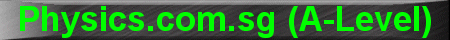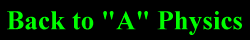(New tips are continually added to these pages.  Check back in a few months' time for more)

TOPIC 15:    Atomic Structure and Radioactivity

Tip 1:

What is Binding Energy?

There are 2 definitions, both of which are acceptable although (1) is more preferred than (2) in recent years:

1.    Binding Energy is the work that needs to be done to completely separate a nucleus into individual nucleons.

2.    Binding Energy is the energy released when protons or neutrons combine to form the nucleus.

Tip 2:

In this chapter, I sometimes break a golden rule.

This golden rule is "When solving all physics problems, all units must be in SI base units:  All Time measurements MUST be in seconds; all Mass measurements in kilograms; and all Length measurements in metres"

However, when calculating the Decay Constant l it is sometimes acceptable to let its unit remain as "hour-1" "day-1" "month-1" or even "year-1" rather than "second-1"

This is because in the radioactive equations:

N = No exp (-lt) and A = Ao exp (-lt)

If we let the time t be in hour/day/month/year, so long as the units for the time (t) matches the reciprocal of the units for the decay constant (l), they will cancel out.  For example, if  l is in "day-1" and t is measured in days, they will cancel out to give a dimensionless constant.

However, do be careful...

When using the equation: A = lN to calculate the Activity in Becquerel (Bq), the Decay Constant l has be in "second-1".  This is because the activity rate (Bq) measures the "number of decays per second".  Not "per hour", "per day", "per month" or "per year"